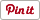Free
Genres:
• Productivity
• Education

# My Script Calculator app review: jot an equation and have it typed properly in seconds

Reviews

## Introduction

My Script Calculator is an iPad app that takes your chicken scratch and gives you the answer. I love this app and use it for my own calculations often. It is the first calculator I downloaded to my iPad, and have kept it handy ever since.

The best iPad apps for calculators

My Script Calculator turns your chicken scratch mathematical equations into an easy to read typed question with the answer. The only thing better would be if it let you solve the question step by step! This app is handy for anyone who is doing more advanced algebra and requires a quick answer. Even if you are making a quilt this app will be helpful to figure out how much material you need!

The best iPhone apps for scientific calculators

If you write with your finger 3 x 3 it will calculate the answer. If you write (21/7) + ✓4  you get that answer too. At first I had trouble drawing the question fast enough, but then found that in the settings you can choose to have it calculate automatically or to press the calculate button. I need the calculate button so I can finish the equations.Example of equation solved with calculate button enabled

## As An Assistive Teaching Tool

My Script Calculator is a great teaching tool because your child can write the question into the app and have the correct answer shown. It does not show the steps for your child to see where they went wrong, but at least it is reliable in processing order of operations. If they typed a question left to right into a regular calculator it would not calculate by order of operations, thus giving the incorrect answer.

While I love this app, I am still on the look out for one that will check your work when you write a question, and then solve. Kids need to see where they went wrong, or they just get frustrated at having to start the question all over.Example of equation

## Pros & Cons

Pros

• Free
• Easy to use, just like paper
• Auto calculate or click the button
• Large area to write in

Cons

• I can't solve my problem and check the answersExample of equation solved

## Final Thoughts

My Script Calculator is an iPad and iPhone app that allows you to jot the question with your finger, and then get an answer. Be it a simple multiplication or an equation, this app will solve your math question. One thing I would like to see, is the ability to have the student solve the question, and then the app check the work. I'm still on the look out for this, however, this app does do proper order of operation versus your student typing the question into a calculator left to right and getting the wrong answer based on not using order of operations. Overall, this app is very helpful, intuitive to use, and easy to correct with write over, scratch out, and more. Definitely recommend it to those working on more advanced math homework.